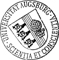## Comparative Study of the Dynamics of Lipid Membrane Phase Decomposition in Experiment and Simulation

• Phase decomposition in lipid membranes has been the subject of numerous investigations both experimentally and by theoretical simulation. Yet quantitative comparisons of the simulated data to the experimental results are rare. In this work we present a novel way of comparing the temporal development of liquid ordered domains obtained from numerically solving the Cahn–Hilliard equation and by inducing phase transition in giant unilamellar vesicles (GUVs). Quantitative comparison is done by calculating the structure factor of the domain pattern. It turns out that the decomposition takes place in three distinct regimes both in experiment and simulation. These regimes are characterized by different rates of growth of the mean domain diameter and there is a quantitative agreement between experiment and simulation as to the duration of each regime and the absolute rate of growth in each regime.

Author: Stefan Burger, Thomas Fraunholz, Christian T. Leirer, Ronald H. W. HoppeGND, Achim WixforthORCiDGND, Malte A. PeterGND, Thomas FrankeGND urn:nbn:de:bvb:384-opus4-21392 https://opus.bibliothek.uni-augsburg.de/opus4/2139 Preprints des Instituts für Mathematik der Universität Augsburg (2012-15) Preprint English Universität Augsburg 2012/11/27 lipid decomposition; Cahn-Hilliard; quantitative comparison Lipidmembran; Phasenumwandlung; Vesikel; Mathematische Modellierung; Numerisches Verfahren; Experiment; Cahn-Hilliard-Gleichung Mathematisch-Naturwissenschaftlich-Technische Fakultät Mathematisch-Naturwissenschaftlich-Technische Fakultät / Institut für Mathematik Mathematisch-Naturwissenschaftlich-Technische Fakultät / Institut für Physik Mathematisch-Naturwissenschaftlich-Technische Fakultät / Institut für Mathematik / Lehr- und Forschungseinheit Angewandte Analysis Mathematisch-Naturwissenschaftlich-Technische Fakultät / Institut für Mathematik / Lehrstuhl für Numerische Mathematik Mathematisch-Naturwissenschaftlich-Technische Fakultät / Institut für Physik / Lehrstuhl für Experimentalphysik I 5 Naturwissenschaften und Mathematik / 51 Mathematik / 510 Mathematik 5 Naturwissenschaften und Mathematik / 53 Physik / 530 PhysikDeutsches Urheberrecht mit Print on Demand